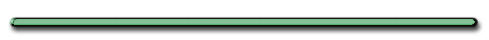AP Physics B

Ever see an object roll UP hill?C. Solving Word Problemsex) A car produces a 92 m skid mark with a deceleration of 7.00 m/s2. What speed was the car travelling just before it applied the brakes?

Vi = ?

a = -7.00 m/s2

Vf = 0

Δd = 92 mVf2 = Vi2 + 2aΔd

0 = Vi2 + 2(-7.00 m/s2)92 m

Vi2 = 1288

Δd = 36 m/s`	`
``

ex) A car accelerates uniformly from rest at a rate of +10 m/s2. How far will the car travel in 4 seconds?

Step 2 - Turn all your information into letters

Vi = 0 (starts from rest)

a = +10 m/s2

t = 4 seconds

d = ?

Step 3 - Substitute and Solve Equation:Δd = Vit + 1/2aΔt2

= 0 m/s(4 sec)+1/2(10 m/s2)(4s)2

Δd = 0 + 5(16)

Δd = 80 m` `
` `Ex) If a car traveling 20 mph can stop in about 40 ft. If the same car was traveling twice as fast, how far would it take to stop?

Vf2 = Vi2 + 2aΔd

0= Vi2 + 2aΔd

-Vi2  = 2aΔd

4 times further

160 ftDisplacement,  Plots